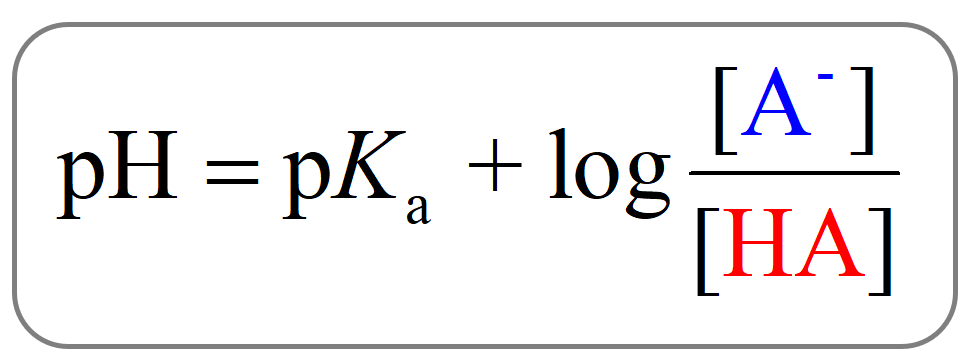## General Chemistry

In the previous post, we talked about the pH of buffer solutions and the two approaches to calculating it.

Let’s now discuss how to prepare a buffer with a given pH. So, we need to choose a proper acid/base pair with certain concentrations. When choosing the acid, remember that the acid should have a pKa as close to the desired pH value as possible.

This can be seen from the Henderson–Hasselbalch equation.When [A] = [HA], pH = pKa since the log term is equal to zero.

For example, if we need a buffer with pH = 4.75, an acid with a pKa close to 4.75 would be a great candidate.

The pKa of acetic acid is 4.75, and if a buffer solution contains, for example , 0.2 M CH3CO2 and 0.2 M CH3CO2H, the pH will be equal to the pKa:

${\rm{pH}}\; = \;{\rm{p}}{K_{\rm{a}}}\;{\rm{ + }}\;{\rm{log}}\frac{{{\rm{[C}}{{\rm{H}}_{\rm{3}}}{\rm{C}}{{\rm{O}}_{\rm{2}}}^{\rm{ – }}{\rm{]}}}}{{{\rm{[C}}{{\rm{H}}_{\rm{3}}}{\rm{C}}{{\rm{O}}_{\rm{2}}}{\rm{H]}}}}$

${\rm{pH}}\; = \;4.75\; + \,\cancel{{{\rm{log}}\frac{{{\rm{0}}{\rm{.2}}\;{\rm{M}}}}{{{\rm{0}}{\rm{.2}}\;{\rm{M}}}}}}\; = \,4.75$

So, how do we determine the ratio of A/HA if the pH is different than the pKa of the acid with the closest value?

For example, let’s say we need a buffer solution with pH = 4.0.

The closest match is going to be the acetate buffer and to determine the [CH3CO2]/[CH3CO2H] ratio required to prepare it, we need to rearrange the Henderson-Hasselbalch equation to obtain an expression for [CH3CO2] and [CH3CO2H]:

${\rm{pH}}\; = \;{\rm{p}}{K_{\rm{a}}}\;{\rm{ + }}\;{\rm{log}}\frac{{{\rm{[C}}{{\rm{H}}_{\rm{3}}}{\rm{C}}{{\rm{O}}_{\rm{2}}}^{\rm{ – }}{\rm{]}}}}{{{\rm{[C}}{{\rm{H}}_{\rm{3}}}{\rm{C}}{{\rm{O}}_{\rm{2}}}{\rm{H]}}}}$

Therefore,

${\rm{log}}\frac{{{\rm{[C}}{{\rm{H}}_{\rm{3}}}{\rm{C}}{{\rm{O}}_{\rm{2}}}{\rm{Na]}}}}{{{\rm{[C}}{{\rm{H}}_{\rm{3}}}{\rm{C}}{{\rm{O}}_{\rm{2}}}{\rm{H]}}}}\;\; = \;{\rm{pH}}\; – \;{\rm{p}}{K_{\rm{a}}}\; = \;4.0\; – \;4.75\; = \; – 0.75$

The antilog of this expression is:

antilog (-0.75) = 10-0.75 = 0.18

${10^{\left( {{\rm{log}}\frac{{[{\rm{C}}{{\rm{H}}_{\rm{3}}}{\rm{C}}{{\rm{O}}_{\rm{2}}}{\rm{Na}}]}}{{[{\rm{C}}{{\rm{H}}_{\rm{3}}}{\rm{C}}{{\rm{O}}_{\rm{2}}}{\rm{H}}]}}} \right)}}\;\; = \;{10^{\left( { – 0.75} \right)}}$

$\frac{{{\rm{[C}}{{\rm{H}}_{\rm{3}}}{\rm{C}}{{\rm{O}}_{\rm{2}}}{\rm{Na]}}}}{{{\rm{[C}}{{\rm{H}}_{\rm{3}}}{\rm{C}}{{\rm{O}}_{\rm{2}}}{\rm{H]}}}}\; = \;0.18$

This means that, for example, if the solution contains 1.0 M CH3CO2H, the concentration of CH3CO2 would have to be 0.18 M.

Check Also